Intro to Probability

Probability is basically the chance of something happening.

You might want to know the chance of it raining today, the chance of getting heads or tails, the chance of rolling an even, the chance of getting picked for something. All of these chances have a numerical value called the probability of the even occurring.

Probabilities are written as fractions, decimals, and percent.

You can determine the fraction and then convert to the decimal or percent form.Check out some examples:

#1. Molly has a bag with 20 marbles. The bag contains 4 green, 8 blue, 5 red and 3 white marbles.

a.  What is the chance of pulling out a blue marble?

Step 1: How many blue marbles are there? 8

Step 2: How many marbles are there total? 20

Step 3: Write as a fraction: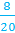Step 4: Simplify the fraction.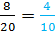Leave the fraction with a denominator of 10 so that you can easily convert to a decimal or percent.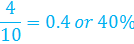There is a 40% chance of pulling a blue marble from the bag.

b.  What is the chance of pulling out a red marble?

Step 1: How many red marbles are there? 5

Step 2: How many marbles are there total? 20

Step 3: Write as a fraction:Step 4: Simplify the fraction.Here we have a unit fraction that may be easier to work with.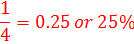There is a 25% chance of pulling a red marble from the bag.

c.  What is the chance of pulling out a green or white marble?

Step 1: How many green and white marbles are there? 7

(Note: When it says "OR" you will add together the two types!)

Step 2: How many marbles are there total? 20

Step 3: Write as a fraction: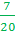Step 4: Convert the fraction to decimal and percent form.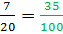Here we have a unit fraction that may be easier to work with.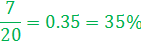There is a 35% chance of pulling a green or white marble from the bag.

Let's try another set of questions.
These questions all deal with a normal number cube or die.a.  What is the chance of rolling a 3?

There is 1 side that is a three out of 6 different sides.b.  What is the chance of rolling a 5?

There is 1 side that is a five out of 6 different sides.c.  What is the chance of rolling an even number?

The evens include 2, 4, and 6. So there are three out of six sides.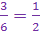d.  What is the chance of rolling a 7?

There are no sevens. When the outcome is not possible,
the probability is zero.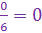e.  What is the chance of rolling a number less than 3?

The numbers less than three include the one and two. So there are 2 possible ways of getting a number less than 3 out of 6 possible sides.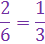f.  What is the chance of rolling a number less than 7?

All of the numbers on the number cube are less than 7. So all 6 sides are possible outcomes.When the probability of something is equal to 1, this is the same as having a 100% chance of the even occurring. Whenever you roll the die, you will ALWAYS get a number less than 7.

Let's Review:

To calculate the probability, write a fraction. The top number is the number of ways the outcome can occur. The bottom number is the number of different outcomes. From the fraction, you can convert to a decimal or a percent. These can also be used to describe a probability.